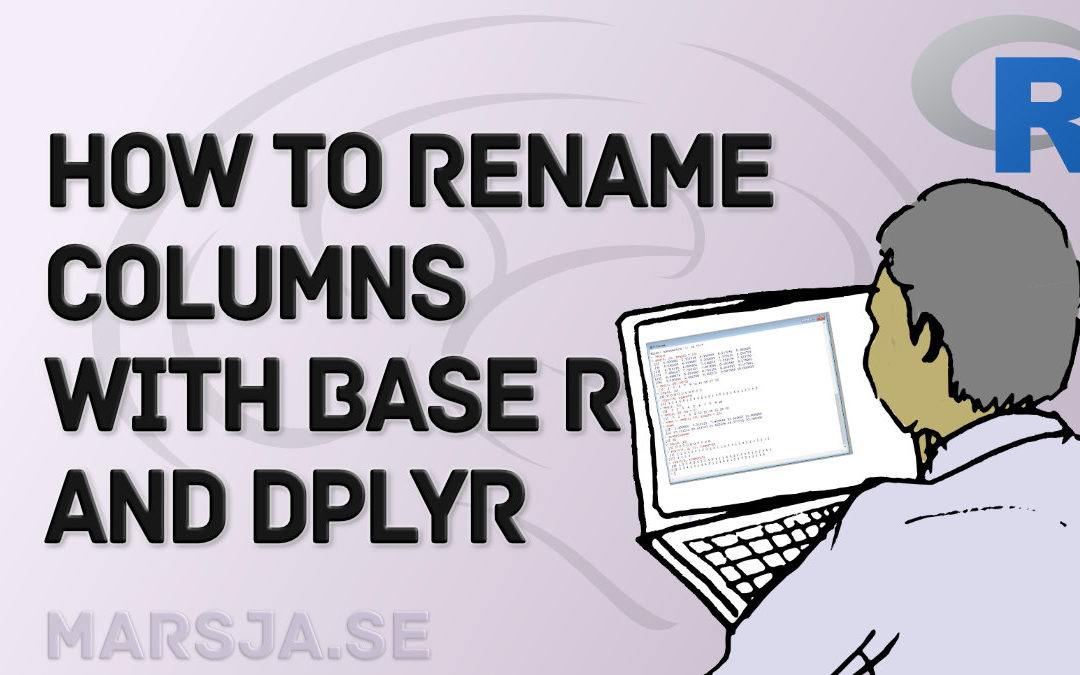## How to Rename Column (or Columns) in R with dplyr

In this data science tutorial, you will learn how to rename a column (or multiple columns) in R using base functions as well as dplyr. Renaming columns in R is a very easy task, especially using the rename() function. Now, renaming a column with dplyr and the rename()...## How to Take Absolute Value in R – vector, matrix, & data frame

In this data science tutorial, you will learn how to get the absolute value in R. Specifically, you will learn how to get the absolute value using the built-in function abs(). As you may already suspect, using abs() is very easy and to take the absolute value from...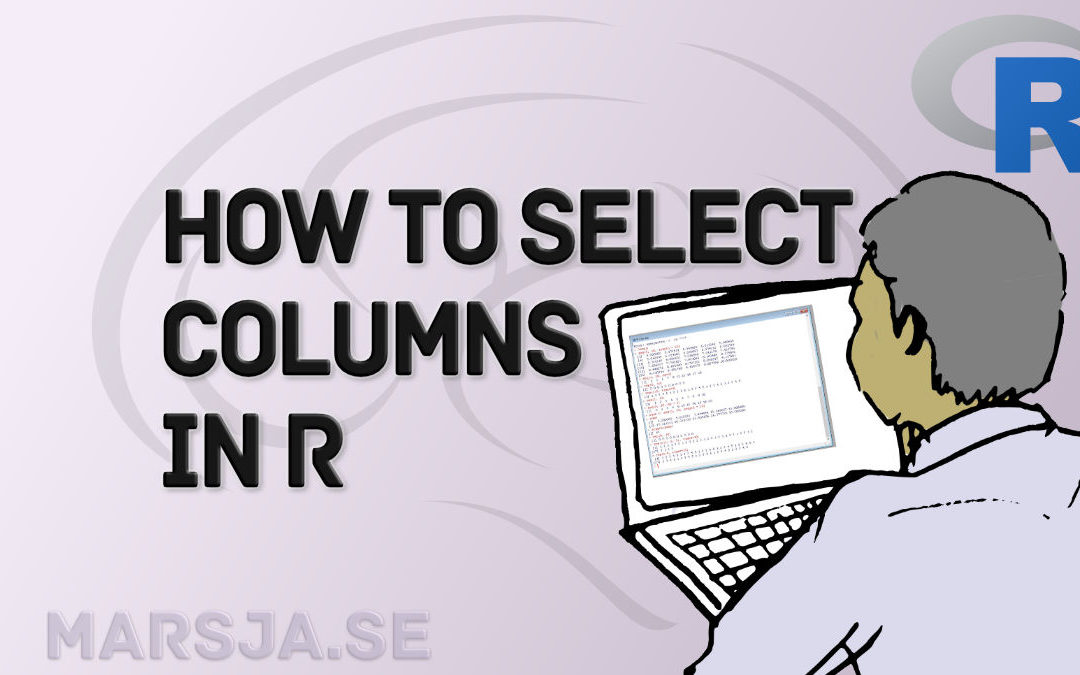## Select Columns in R by Name, Index, Letters, & Certain Words with dplyr

In this R tutorial, you will learn how to select columns in a dataframe. First, we will use base R, in a number of examples, to choose certain columns. Second, we will use dplyr to get columns from the dataframe. Outline In the first section, we are going to have a...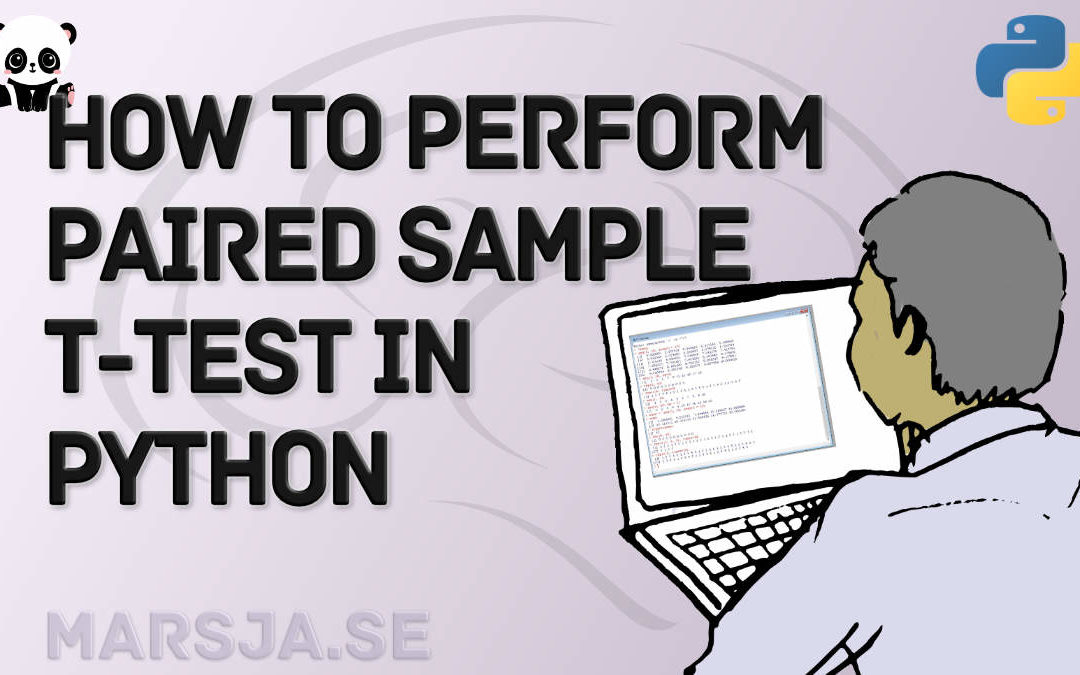## How to use Python to Perform a Paired Sample T-test

In this Python data analysis tutorial, you will learn how to perform a paired sample t-test in Python. First, you will learn about this type of t-test (e.g. when to use it, the assumptions of the test). Second, you will learn how to check whether your data follow the...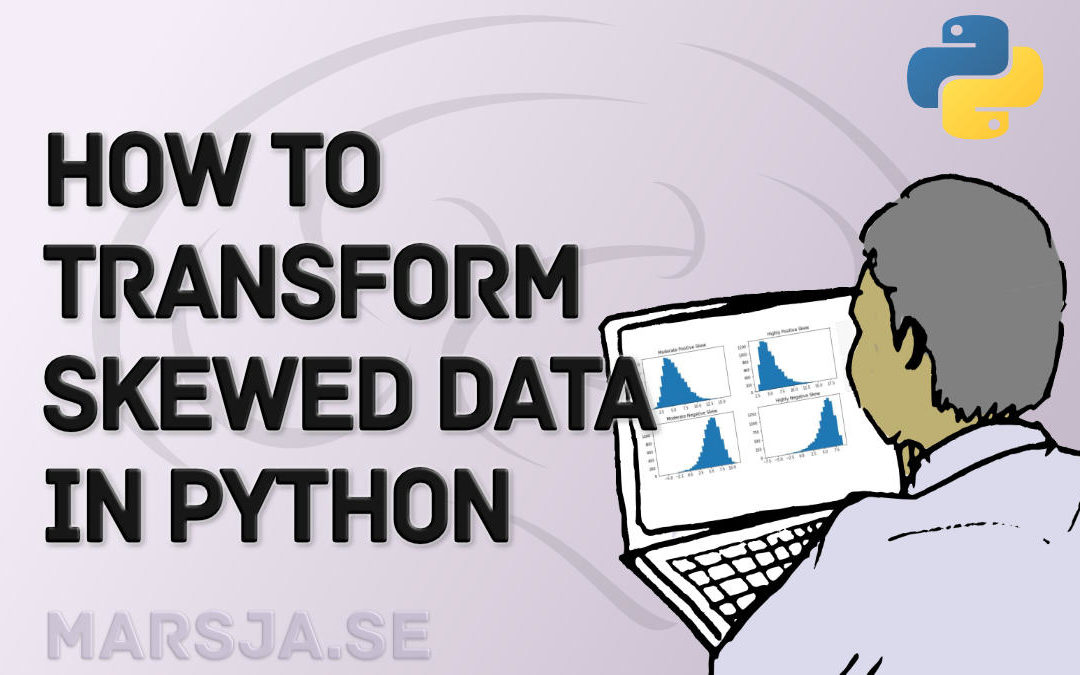## How to use Square Root, log, & Box-Cox Transformation in Python

In this tutorial, related to data analysis in Python, you will learn how to deal with your data when it is not following the normal distribution. One way to deal with non-normal data is to transform your data. In this post, you will learn how to carry out Box-Cox,...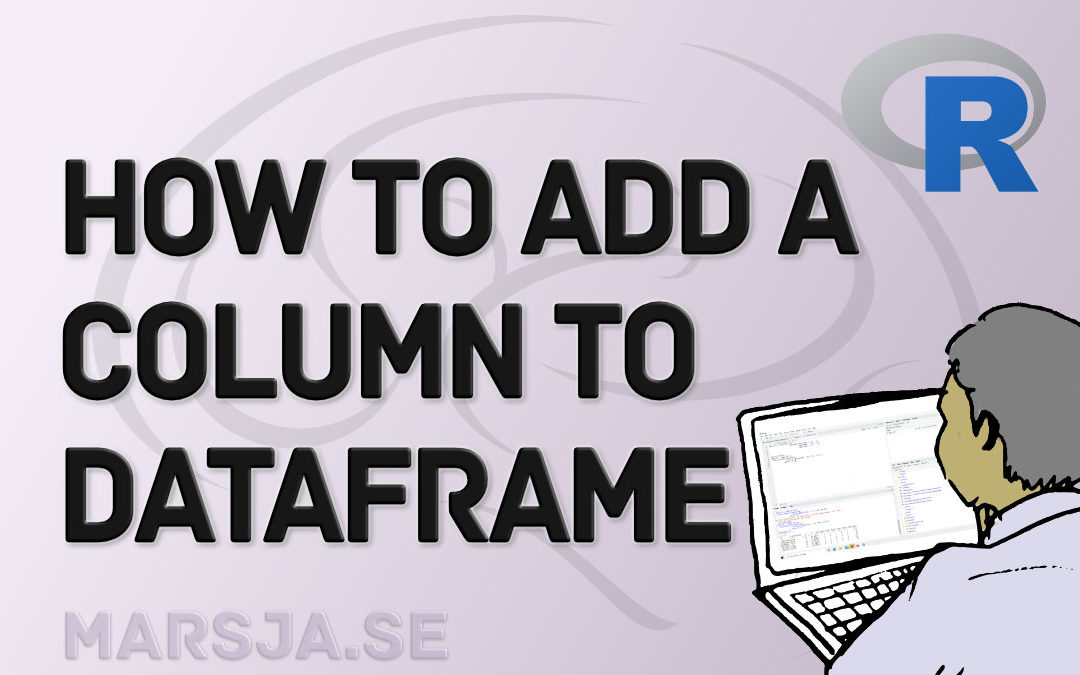## How to Add a Column to a Dataframe in R with tibble & dplyr

In this brief tutorial, you will learn how to add a column to a dataframe in R. More specifically, you will learn 1) to add a column using base R (i.e., by using the \$-operator and brackets, 2) add a column using the add_column() function (i.e., from tibble), 3) add...